-->

# Ultimate C++ Programming Language Cheat Sheet

## C++ Programming Language Cheat Sheet

What is C++?
C++  is a general-purpose programming language. It has imperative, object-oriented and generic programming features, while also providing facilities for low-level memory manipulation. It was designed with a bias toward system programming and embedded, resource-constrained and large systems, with performance, efficiency and flexibility of use as its design highlights.

Data Types
Set of values together with a set of operations called data type.C++ data types classified into three categories:
1.Simple data type
2.Structured data type
3.Pointers

Integral Data Types
1. char
2. short
3. int
4. long
5. bool
6. unsigned char
7. unsigned short
8. unsigned int
9. unsigned long
Floating-Point Data Types :
1. float
2. double
3. long double

# Standard Library

The C++ Standard Library can be categorized into two parts:
• The Standard Function Library: This library consists of general-purpose, stand-alone functions that are not part of any class. The function library is inherited from C.
• The Object-Oriented Class Library: This is a collection of classes and associated functions.

# Variable Types

TypeDescription
boolStores either value true or false.
charTypically a single octet(one byte). This is an integer type.
intThe most natural size of the integer for the machine.
floatA single-precision floating point value.
doubleA double-precision floating point value.
voidRepresents the absence of type.

Operators in C++
1. Arithmetic Operators
2. Relational Operators
3. Logical Operators
4. Bitwise Operators
5. Assignment Operators
6. Misc Operators
1.Arithmetic Operators.
OperatorDescriptionExample
+Adds two operandsX + Y = 20
Subtracts second operand from the firstX – Y = 8
*Multiplies both operandsX * Y = 84
/Divides numerator by de-numeratorX / Y = 2
%Modulus Operator and remainder of after an integer divisionY % X= 0
++Increment operator, increases integer value by oneX++ = 15
Decrement operator, decreases integer value by oneX– =  13

## II.Relational Operators

OperatorDescriptionExample
==Checks if the values of two operands are equal or not. If yes, then the condition becomes true.(X == Y) is not true.
!=Checks if the values of two operands are equal or not. If the values are not equal, then the condition becomes true.(X != Y) is true.
>Checks if the value of left operand is greater than the value of the right operand. If yes, then the condition becomes true.(X > Y) is not true.
<Checks if the value of left operand is less than the value of the right operand. If yes, then the condition becomes true.(X < Y) is true.
>=Checks if the value of left operand is greater than or equal to the value of the right operand. If yes, then the condition becomes true.(X >= Y) is not true.
<=Checks if the value of left operand is less than or equal to the value of the right operand. If yes, then the condition becomes true.(X <= Y) is true

## III.Logical Operators

OperatorDescriptionExample
&&Called Logical AND operator. If both the operands are non-zero, then the condition becomes true.(X && Y) is false.
||Called Logical OR Operator. If any of the two operands is non-zero, then the condition becomes true.(X || Y) is true.
!Called Logical NOT Operator. It is used to reverse the logical state of its operand. If a condition is true, then Logical NOT operator will make it false.!(X && Y) is true.

## IV. Bit-wise Operators

OperatorDescriptionExample
&Binary AND Operator copy a bit to the result if it exists in both operands.(X &Y) = 12
|Binary OR Operator copies a bit if it exists in either operand.(X | Y) = 61
^Binary XOR Operator copies the bit if it is set in one operand but not both.(X ^ Y) = 49
~Binary One's Complement Operator is unary and has the effect of ‘flipping’ bits.(~X ) = -211,
<<Binary Left Shift Operator. The left operands value is moved left by the number of bits specified by the right operand.X << 2 = 200
>>Binary Right Shift Operator. The left operands value is moved right by the number of bits specified by the right operand.X >> 2 = 19

## V.Assignment Operators

OperatorDescriptionExample
=Simple assignment operator. Assigns values from right side operands to left side operandZ = X + Y will assign the value of X + Y to Z
+=Add AND assignment operator. It adds the right operand to the left operand and assigns the result to the left operand.Z += X is equivalent to Z = Z + X
-=Subtract AND assignment operator. It subtracts the right operand from the left operand and assigns the result to the left operand.Z -= X is equivalent to Z = Z – X
*=Multiply AND assignment operator. It multiplies the right operand with the left operand and assigns the result to the left operand.Z *= X is equivalent to Z = Z * X
/=Divide AND assignment operator. It divides the left operand with the right operand and assigns the result to the left operand.Z /= X is equivalent to Z = Z/ X
%=Modulus AND assignment operator. It takes modulus using two operands and assigns the result to the left operand.Z %= X is equivalent to Z = Z % X
<<=Left shift AND assignment operator.Z <<= 2 is same as Z = Z << 2
>>=Right shift AND assignment operator.Z >>= 2 is same as Z = Z >> 2
&=Bitwise AND assignment operator.Z &= 2 is same as Z = Z & 2
^=Bitwise exclusive OR and assignment operator.Z ^= 2 is same as Z = Z ^ 2
|=Bitwise inclusive OR and assignment operator.Z |= 2 is same as Z = Z | 2

## VI.Misc Operators

OperatorDescriptionExample
sizeof()Returns the size of a variable.sizeof(x), where a is an integer, will return 4.
&Returns the address of a variable.&X; returns the actual address of the variable.
*Pointer to a variable.*X;
? :Conditional Expression.If Condition is true? then value X: otherwise value Y

# Standard I/O Devices

• Use iostream to extract (receive) data from keyboard and send output to the screen
• IOstream contains definitions of two types:

1. istream – input stream
2. ostream – output stream

• Basic IOstream variables:

1. cin – common input
2. cout – common output

# cin and cout

To use cin and cout, the preprocessor directive #include <iostream> must be used
The declaration is similar to the following C++ statements:
1. istream cin;
2. ostream cout;
Input stream variables: type istream
Output stream variables: type ostream
FUNCTIONS
What are Functions?
function is a group of statements that together perform a task. Every C program has at least one function, which is main(), and all the most trivial programs can define additional functions. You can divide up your code into separate functions.

# Predefined and User-Defined Functions

1) Predefined standard library functions – These are available to anyone who writes a C++ program. This saves the programmer’s time from writing own functions. They are completely debugged, efficient and always produce a precise output. The important contribution of the system-supplied functions is that they provide clarity to the program because they do not have to be redefined. It reduces the source code, which saves the time of the programmer.
2) User Defined functions – C++ language allows additional functions besides the built-in functions called the user-defined function. It allows programmers to define their own functions. The programmer must code the logic of this type. In order to do so, a declaration is needed.

# Enumeration Data Type

An enumeration is a distinct type whose value is restricted to a range of values (see below for details), which may include several explicitly named constants (“enumerators“). The values of the constants are values of an integral type known as the underlying type of the enumeration.
Data types:
C++ simple data types:
1. integral (char, short, int, long, and bool)
2. enum
3. floating (float, double, long double)
C++ structured data types:
1. array
2. struct
3. union
4. class

# Arrays

C++ provides a data structure, the array, which stores a fixed-size sequential collection of elements of the same type. An array is used to store a collection of data, but it is often more useful to think of an array as a collection of variables of the same type.

## C++ Arrays in Detail

ConceptDescription
Multi-dimensional arraysC++ supports multidimensional arrays. The simplest form of the multidimensional array is the two-dimensional array.
Pointer to an arrayYou can generate a pointer to the first element of an array by simply specifying the array name, without any index.
Passing arrays to functionsYou can pass to the function a pointer to an array by specifying the array’s name without an index.
Return array from functionsC++ allows a function to return an array.

# Structures

The structure is a collection of variables of different data types under a single name. It is similar to a class in that, both hold a collection of data of different data types.

## How to define a structure variable?

Once you declare a structure person as above. You can define a structure variable as:

## How to define a structure variable?

Once you declare a structure person as above.
Person Jack;
Here, a structure variable Jack is defined which is of type structure person.
When structure variable is defined, only then the required memory is allocated by the compiler.

## How to access members of a structure?

The members of structure variable are accessed using a dot (.) operator.
Suppose, you want to access salary of structure variable Jack and assign it 98000 to it.
Jack.age=98000;

# Strings

S.N.Function & Purpose
1strcpy(s1, s2);Copies string s2 into string s1.
2strcat(s1, s2);Concatenates string s2 onto the end of string s1.
3strlen(s1);Returns the length of string s1.
4strcmp(s1, s2);Returns 0 if s1 and s2 are the same; less than 0 if s1<s2; greater than 0 if s1>s2.
5strchr(s1, ch);Returns a pointer to the first occurrence of character ch in string s1.
6strstr(s1, s2);Returns a pointer to the first occurrence of string s2 in string s1.

## The String Class in C++

The standard C++ library provides a string class type that supports all the operations mentioned above, additionally much more functionality. We will study this class in C++ Standard Library

# Pointers

## What Are Pointers?

pointer is a variable whose value is the address of another variable. Like any variable or constant, you must declare a pointer before you can work with it.

## Using Pointers in C++:

There are few important operations, which we will do with the pointers very frequently. (x) we define a pointer variable (y)assign the address of a variable to a pointer and (z) finally access the value at the address available in the pointer variable. This is done by using unary operator * that returns the value of the variable located at the address specified by its operand.

## C++ Pointers in Detail

ConceptDescription
C++ Null PointersC++ supports null pointer, which is a constant with a value of zero defined in several standard libraries.
C++ pointer arithmeticThere are four arithmetic operators that can be used on pointers: ++, –, +, –
C++ pointers vs arraysThere is a close relationship between pointers and arrays. Let us check how?
C++ array of pointersYou can define arrays to hold a number of pointers.
C++ pointer to pointerC++ allows you to have a pointer to a pointer and so on.
Passing pointers to functionsPassing an argument by reference or by address both enable the passed argument to be changed in the calling function by the called function.
Return pointer from functionsC++ allows a function to return a pointer to local variable, static variable and dynamically allocated memory as well.

## I/O Library Header Files

Header FileFunction and Description
<iostream>This file defines the cin, cout, cerr and clog objects, which correspond to the standard input stream, the standard output stream, the un-buffered standard error stream and the buffered standard error stream, respectively.
<iomanip>This file declares services useful for performing formatted I/O with so-called parameterized stream manipulators, such as setw and setprecision.
<fstream>This file declares services for user-controlled file processing. We will discuss it in detail in File and Stream related chapter.

# Inheritance

Derive quality and characteristics from parents or ancestors. Like you inherit features of your parents.
Types of Inheritance:
1. Single Inheritance.
2. Multilevel  Inheritance.
3. Multiple Inheritance.
4. Hierarchical  Inheritance.
5. Hybrid  Inheritance.
Polymorphism
Polymorphism is one of the crucial features of c++ it simply means one name and multiple forms.The function overloading and operator overloading is known as compile time Polymorphism / static Polymorphism.

# Data Abstraction

Data abstraction refers to, providing only essential information to the outside world and hiding their background details, i.e., to represent the needed information in program without presenting the details.Data abstraction is a programming (and design) technique that relies on the separation of interface and implementation.

# Data Encapsulation

Data encapsulation is a mechanism of bundling the data, and the functions that use them and data abstraction is a mechanism for exposing only the interfaces and hiding the implementation details from the user.C++ supports the properties of encapsulation and data hiding through the creation of user-defined types, called classes. We already have studied that a class can contain private, protected and public members.

#### 0 comments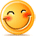:)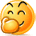:-)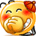:))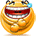=))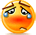:(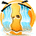:-(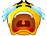:((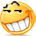:d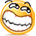:-d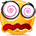@-)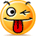:p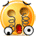:o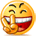:>)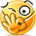(o)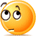[-(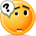:-?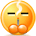(p)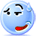:-s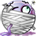(m)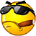8-)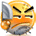:-t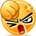:-b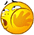b-(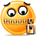:-#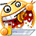=p~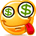:-\$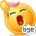(b)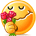(f)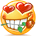x-)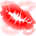(k)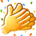(h)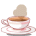(c)cheer

Posts RSSComments RSSBack to top
© 2013 ComboUpdates - Powered by Blogger
Released under Creative Commons 3.0 CC BY-NC 3.0